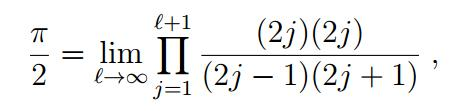News Release

# Discovery of classic pi formula a 'cunning piece of magic'

Wallis formula buried in a quantum mechanics calculation

Peer-Reviewed Publication

University of Rochesterimage: Figure 1: Calculations derived by Tamar Friedmann and Carl Hagen resulted in an expression of special mathematical functions called gamma functions. view more  Credit: Tamar Friedmann and Carl Hagen/University of Rochester

While most people associate the mathematical constant π (pi) with arcs and circles, mathematicians are accustomed to seeing it in a variety of fields. But two University of Rochester scientists were still surprised to find it lurking in a quantum mechanics formula for the energy states of the hydrogen atom.

"We didn't just find pi," said Tamar Friedmann, a visiting assistant professor of mathematics and a research associate of high energy physics, and co-author of a paper published this week in the Journal of Mathematical Physics. "We found the classic 17th century Wallis formula for pi, making us the first to derive it from physics, in general, and quantum mechanics, in particular."

The Wallis formula--developed by British mathematician John Wallis in his book Arithmetica Infinitorum--defines π as the product of an infinite string of ratios made up of integers. For Friedmann, discovering the Wallis formula for π in a quantum mechanics formula for the hydrogen atom's energy states underscores π's omnipresence in math and science.

"The value of pi has taken on a mythical status, in part, because it's impossible to write it down with 100 percent accuracy," said Friedmann, "It cannot even be accurately expressed as a ratio of integers, and is, instead, best represented as a formula."

Friedmann did not set out to look for π nor for the Wallis formula. The discovery began in a quantum mechanics course taught by Carl Hagen, a professor of physics at the University of Rochester and one of the six physicists who predicted the existence of the Higgs boson. While the quantum calculations developed by Danish physicist Niels Bohr in the early 20th century give accurate values for the energy states of hydrogen, Hagen wanted his students to use an alternate method--called the variational principle--to approximate the value for the ground state of the hydrogen atom. Like the Wallis formula, the variational principle dates back to the 17th century, one of its first appearances being the Principle of Least Time of mathematician Pierre de Fermat, a contemporary of Wallis. Hagen also started thinking about whether it would be possible to apply this method to states other than the ground state. Hagen got Friedmann involved to take advantage of her ability to work in both physics and mathematics.

Although applying the variational principle to calculate the ground state of a hydrogen atom is a relatively straightforward problem, its applicability to an excited state is far from obvious. This is because the variational principle cannot ordinarily be applied if there are lower energy levels. However, Friedmann and Hagen were able to get around that by separating the problem into a series of l problems, each of which focused on the lowest energy level for a given orbital angular momentum quantum number, l.

They could then calculate the values for the different energy states and compare them with the values obtained by Bohr almost a century ago. This enabled them to determine how the ratio of the Bohr values to the values obtained with the 'tweaked' variational principle changed as higher and higher energy levels were taken into account. And they were surprised to see that the ratio yielded--effectively--the Wallis formula for π.

Specifically, the calculation of Friedmann and Hagen resulted in an expression involving special mathematical functions called gamma functions, leading to a formula which could be reduced to the classic Wallis formula (See figures).

"What surprised me is that the formula occurred in such a natural way in the calculations, with no circles involved in determining the energy states," said Hagen, the co-author of the paper. "And I am glad I didn't think about this before Tamar arrived in Rochester, because it would have gone nowhere and we would not have made this discovery."

Mathematician Moshe Machover of King's College London calls the finding a "cunning piece of magic."

"This derivation of pi is a surprise of the familiar, much like a magician's trick," said Machover. "A child who sees a trick done for the first time may be only surprised. But an adult, who has seen numerous tricks over the years, experiences both surprise and familiarity."

Addressing the centuries-long gap between the 17th century Wallis formula, the 20th century quantum theory, and the decades that passed from that time to now, Doug Ravenel, a professor of mathematics at the University of Rochester, points out that Friedmann and Hagen used long-established concepts of their fields to arrive at their result, so even mathematicians and physicists who lived many decades ago would have been able to appreciate it.

"This is a beautiful connection between pi and quantum mechanics that could have been found 80 years ago, but was not discovered until now," said Ravenel, congratulating the two authors.

While it took nearly a century to discover this classical-quantum connection, getting it published took far less time; the Journal of Mathematical Physics accepted the paper in less than 24 hours.

###

Disclaimer: AAAS and EurekAlert! are not responsible for the accuracy of news releases posted to EurekAlert! by contributing institutions or for the use of any information through the EurekAlert system.